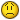# Subtotal data excluding negitive numbers.

T

#### Tony

some other spreadsheet. In column “A” some numbers are positive and
some are negative. What I want to do is to subtotal(9,A3:A100) to show
the total numbers when I filter something out. While I don’t want
these negative numbers to be calculated. I knew if I add a new column
can resolve this problem, but if I the spreadsheet does not allow me
to do that change, how can I put kind of formula in one single cell to
show the subtotal result without adding the negative numbers?

I have tried sumproduct but result only showing the full sum whatever
how you filter it out.

Thanks.

Z

#### zvkmpw

In column “A” some numbers are positive and
some are negative. What I want to do is to subtotal(9,A3:A100) to show
the total numbers when I filter something out.
... how can I put kind of formula in one single cell to
show the subtotal result without adding the negative numbers?

One way is to use something like
=SUMIF(\$A:\$A,">0")

Another way is to auto-filter column A, choosing
Custom > is greater than > 0
and
=SUBTOTAL(109,\$A:\$A)
The code 109 is like 9, but it "ignores hidden values" (ref.: Excel's
built-in Help).

T

#### Tom Hutchins

Try

=SUMPRODUCT(--(A3:A100>0),SUBTOTAL(9,OFFSET(A3:A100,ROW(A3:A100)-ROW(A3),0,1)))

Hope this helps,

Hutch

T

#### Tony

Try

=SUMPRODUCT(--(A3:A100>0),SUBTOTAL(9,OFFSET(A3:A100,ROW(A3:A100)-ROW(A3),0,­1)))

Hope this helps,

Hutch

- Show quoted text -

Seems it's not working ...